﻿ ARCH Model in Analysis Patterns of Rupiah Exchange Rates against US DollarPublications are Open
Access in this journal
Article Versions
Export Article
• Normal Style
• MLA Style
• APA Style
• Chicago Style
Open Access Peer-reviewed

### ARCH Model in Analysis Patterns of Rupiah Exchange Rates against US Dollar

Journal of Finance and Economics. 2020, 8(2), 61-68. DOI: 10.12691/jfe-8-2-3
Received February 11, 2020; Revised March 20, 2020; Accepted April 05, 2020

### Abstract

The Indonesian government uses the exchange rate of US dollar (USD) as an indicator in the preparation of the Draft of State Revenue and Expenditure Budget (RAPBN). The reason is that the daily exchange rate of the US dollar is a time series data with significant autocorrelation. Although the variance is relatively small, it cannot be considered constant. So, that the functional relationship model of the variants needs to be examined in addition to the functional relationship model of the exchange value. The study of the functional relationship model of observational values and their variants can simultaneously be analyzed by ARCH model based on daily data between 1 July 2015 - 31 July 2019.

### 1. Introduction

The assumption of the exchange rate of the Rupiah against the US dollar is one of the important macro indicators in the preparation of Draft of State Revenue and Expenditure Budget (RAPBN) because this exchange rate greatly influences the revenue, expenditure and financing of the RAPBN. Longmore and Robinson (2004) stated the importance of understanding exchange rate behavior with the aim of designing appropriate monetary policy 1. Basic macroeconomic assumptions used as a reference in the 2020 Draft State Budget, namely: 1) Economic growth is expected to continue to grow by 5.3%; 2) Inflation can still be controlled in the range of 3.1%; 3) The Rupiah exchange rate is estimated to be at Rp. 14,400 per US dollar; 4) The interest rate of the 3-month Treasury Bill (SPN) is 5.4% with optimism related to positive perceptions and improvement of the investment climate in Indonesia so that investment flows will continue to flow into the country; 5) Indonesia Crude Price (ICP) is estimated to reach US \$ 65.0 per barrel on average; 6) Oil and gas lifting is estimated to reach 734 thousand barrels per day and 1,191 thousand barrels of oil equivalent per day 2. Therefore, forecasting in the decision making process in the financial sector is very important because it can provide a basis for better planning and decision making 3.

In Indonesia, the exchange rate is influenced by world economic conditions, domestic economic and political conditions. In the era of globalization and financial liberalization, exchange rates play an important role in international trade and finance for a small open economy like Pakistan 4. Global financial markets are influenced by forecast exchange rate volatility. Macroeconomics such as oil prices, interest rates, wages, unemployment, and economic growth rates are significantly influenced by exchange rate forecasting 5; Foreign exchange rates are related to other currencies and fluctuate in the context of demand and supply of currencies 6. Foreign exchange rates, especially US dollar, are serial processes that do not correlate with averages and variances are not constant for certain intervals. In the time series analysis, if the average calculation is not constant, it could be constant through the differential process. But the variance is difficult to be constant, it could only be reduced with the process of variance stability. So to build a model of the exchange rate of the Rupiah against the US dollar must use the ARCH model because in this modeling process the condition of the analyzed data variance is not constant, but the variance in the forecast value is constant.

At the end of the background explanation, the problem that will be solved in this research is to build a model of the exchange rate of the Rupiah against the US dollar using the ARCH process. Because this exchange rate is an uncorrelated serial process, with a mean that can be constant, but the variance cannot. So, in this case, the conditional variance is not constant for observations, but the variance is not conditionally constant for the forecast value.

### 2. Theoretical Framework

2.1. Autoregressive Conditional Heteroscedasticity (ARCH)

In a seminal paper by Engle stated that ARCH was triggered by the most active and useful field of econometric research since the last two decades. The ARCH / GARCH class model successfully captures the well-documented volatility groupings in financial markets 7; The ARCH model allows series variations depending on the set of information available, including time 8; Engle (1982) initially developed the ARCH model to illustrate the ING inflation uncertainty. Then the ARCH class model is used very widely in characterizing the volatility of financial markets that varies over time 9; The ARCH model is a new tool for measuring risk and its impact on returns. They also provide new tools for pricing and hedging non-linear assets such as options. The ARCH model has been developed to measure the price of risk. Another topic for the ARCH model is its usefulness in trading options. Initially it was suspected that the volatility model could provide an indication of mispricing in the options market leading to trading opportunities 10; ARCH model to analyze a series of empirical financial time returns in market. The model to explain its regularity 11; Bera and Higgins state that the main benefit of ARCH is the finding of tangible changes in the volatility of the economic time series that can be predicted and resulted from certain types of nonlinear dependence rather than exogenous structural changes in variables 12; They state that the ARCH model is simple and easy to handle, can handle clustered errors, take care of nonlinearities, and handle changes in econometric expert estimates 12; All ARCH models discussed are univariate. However, assets and markets influence each other not only in terms of expected returns but also in terms of volatility 13; In analyzing financial time series, specifically analyzing the risk of holding assets, evaluating the price of an option, predicting time-varying confidence intervals and obtaining a more efficient time estimator using the Autoregressive Conditional Heteroscedasticity (ARCH) model 13; The recent development of the ARCH literature is to introduce a class of ARCH power models that allows the term free power rather than assuming absolute or quadratic terms in their specifications 14; ARCH, which was discovered in 1982 by Engle, was the first model to assume volatility that is not constant. The GARCH, EGARCH, PARCH and TARCH models are the result of more sophisticated ARCH transformations and developments 15; According to the ARCH model, the variant of the current error term is a function of the term error of the previous time period. Generalizing ARCH models is a solution to avoid the problem of estimating negative variance parameters 15. Engle 16 offers the use of an ARCH model that allows conditional variance to change over time as a function of past errors leaving variances without constant conditions 17; The ARCH and GARCH models relate to data that has heteroscedasticity which uses it in the exact error variant to get a more efficient estimator 18.Non-linear models that have proven to be very useful in finance are autoregressive conditional heteroscedasticity (ARCH) and general autoregressive conditional heteroscedasticity (GARCH) are (18); ARCH and GARCH are used to find out the real exchange rate index 5.

2.2. Methodology

The purpose of this study is to build a Rupiah exchange rate model against the US dollar using the ARCH process. To obtain a model of forecasting the exchange rate of the Rupiah against the US dollar and examining extreme exchange rate patterns. This research is expected to have benefits as a way to develop theories about Time Series and obtain findings of the function and role of Time Series theory on the issue of forecasting foreign exchange rates. It can also improve competence and professionalism for researchers. In the field of Financial Mathematics, this research can expand the literature on forecasting foreign exchange rates on the Rupiah. The purpose of this study is to examine the pattern of time series data on the exchange rate of the Rupiah on US dollars and to build a time series regression model of foreign exchange rates, in this case, US dollars. To get an exchange rate estimation model one week ahead. Time series data is data that is a function of time, so in the time series data is defined autocorrelation. The conception of autocorrelation is identical to the Pearson correlation, which is a correlation between paired quantitative data. In autocorrelation paired data are built based on data lag. For example x1, x2,. . . , xn; time-series data. Autocorrelation lag-k, k = 1, 2 ,. . . << n, is...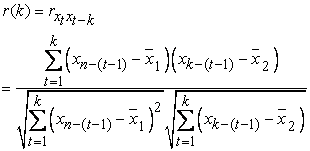(1)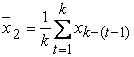Because in the analysis the value of k must be smaller compared to n, so it can be considered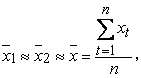and Equation (1) becomes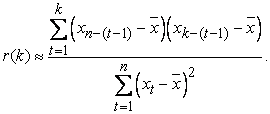(2)

Another conception in time series data is partial autocorrelation, which is autocorrelation of Xt with Xt + k, assuming xt + 1, xt + 2,. . . , xt + (k-1) is constant. To calculate partial autocorrelation and partial autocorrelation, we can use computer software packages, such as SPSS, Statistica and Minitab. Autocorrelation and partial autocorrelation are respectively a function of the lag, and the graph of the lag is called a correlogram which can be used to examine the significance of autocorrelation. If the autocorrelation correlogram constructs a decreasing pattern as the lag value increases, then autocorrelation is significant, but if it constructs a random pattern, the autocorrelation is insignificant. If autocorrelation is significant, the analysis that must be done is time-series data regression analysis, which is regression analysis between data. But when it is not significant, the analysis used is ordinary regression analysis with time as the independent variable and data as the dependent variable. If the time series data has a significant autocorrelation in lag-k, then the time series data regression model is lag-k autoregression, with the equation.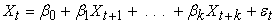(3)

with β0, β1 , . . . , βk parameterr and εt error

Based on a time series data sample, parameter values and errors can be estimated. The assessment can use the SPSS or Statistica program. In the time series data regression analysis, one of the assumptions that must be met is the homogeneity of the variance of the model error and the stationarity of the data that can be analyzed with partial autocorrelation correlograms. If the partial autocorrelation correlogram is cut off in lag-2, then the data is not stationary, which can be stationary with the differential process. If it is not homogeneous (not stationary variance), then two things can be done. The first is to transform the stability of variance, and if this is not possible because it will violate or disrupt science, then the analysis that must be done, in addition to examining the functional relationship between observations (autoregression), also must be examined the model of the error variance (heteroscedasticity).

The condition of variance homogeneity that should not be done is the transformation of variance stability which is often found in cross-section data, for example in modern financial problems. This happens because of the many influence variables that cause uncertainty and the emergence of the desired data value. For example, foreign exchange rates, especially the US dollar. Variables that can cause fluctuations in exchange rates include, people's "tastes" towards foreign currencies, export values, inflation, interest rates, investment, and economic growth. So that the time series data regression analysis that must be done in problems like this is ARCH. Time series of exchange rates often use ARCH family models 19.

### 3. Methods

ARCH Model

The basic model of time series data regression is AR (k) (Auto Regressive lag k), which is a model of the functional relationship between observations at time t, xt, with the previous observations k, xt-1, xt-2,. . . xt-k. The mathematical model of AR (k) is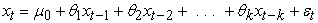(4)

εt model error, commonly called white noise, and assumed E(εt) = 0,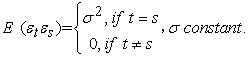With this assumption, the parameter estimate i, i = 0, 1, . . . , k, can be done using the least-squares method, which estimates are statistically unbiased and of minimum variance. The k-order auto-regression model, AR (k), has an inversion model called the Moving Average Model order-p, MA (p), and is an observational functional relationship at time t, xt, with an error at time t, xt, with an error at time t , εt, and the previous error p, εt -1, εt -2, . . . εt -p. The mathematical model is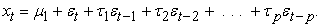(5)

In this connection, the order values AR, k, with MA, p, there is no formulation of functional relations, only on their use, p <k. If the AR (k) model parameter estimation can be used as an estimation method such as the bias regression, then on the MA (p) model must be used the iteration method. At this time, a lot of computer software for calculation in estimating these parameters, for example, SPSS, STATISTICA, and SAS. Because the AR (k) and MA (p) models are two models that are mutually inverted, they can be combined, summed up, and the model is called ARMA (k, p), Autoregressive Moving Average (k, p), the equation is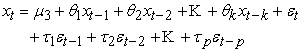(6)

From the formulation of the assumption E(εtεs) =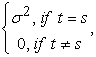it is written that the value of the variance error is a function of time, and εt2 can be presented in the modelεt2 = µ4 + ϕ1εt-12 + ϕ2εt-22 + . . . + ϕkεt-k2 + et..

Assuming E(et) = 0, E(etes) =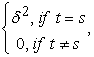δ constant.

This equation is called the ARCH lag k model, written ARCH (k). This model can be used to predict the variance of errors for data that are not too fluctuating so that the process of differential fluctuation can be muted, or in other words, the data becomes stationary. In general, the ARCH (k) formulation model is,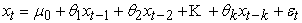(7a)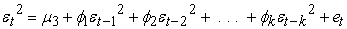(7b)

Assuming E(εtεs) =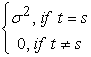and E(et) = 0, E(etes) =σ and δ constant.

If equations (7a) and (7b) are combined, then the equation is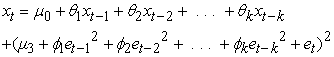(8)

From this formulation, it can be seen that the ARCH (k) model is a function composed of the MA (k) model on the AR (k) model, so statistically the ARCH (k) model is a conditional ARMA (k, k) model.

ARCH Process

In general, time series regression analysis begins with mapping data over time. The aim is to examine the existence of trend components and seasonal components (for monthly data), with data variance patterns. If a trend and/or seasonal component exists, it must be eliminated through a differential process. The second step is to describe ACF and PACF. ACF images are used to examine the significance of autocorrelation and estimated lag values for the ARCH model, while PACF is to examine the order of the differential process. After the stationary data and lag values are determined, the third step is to build the ARCH (k) model and estimate the parameters. Based on Equations (7a) and (7b), the estimation process is:

1. Estimate the parameters in Equation (7a), based on the least-squares method.

2. Identifying the model, to examine the significance of the model, the consistency of model errors and the existence of influential outliers.

3. After the goodness and fit model, do the modeling of the residual squares, and estimate the parameters.

4. Repeat step-2 for the model obtained in step-3.

ARCH Model for Exchange Rates of US Dollar towards Indonesian Rupiah

The exchange rate of foreign exchange on the Rupiah, especially the US dollar, is one indicator in the preparation of the Draft of State Revenue and Expenditure Budget (RAPBN) 20. The value can change every day. This change is caused by global and domestic economic conditions, as well as by socio-political conditions. Abbas Hasbalrasol and Ahmed Mohammed state that in exchange rates affect companies in a country differently, for example, fluctuations caused by oil price reductions due to the global economic crisis 21. So that, foreign exchange rates are time series data which, if examined from changes in value, are non-stationary data on calculated rates and variances 22. The ARCH model for exchange rates was first applied by Hsieh (1998) testing daily data for five exchange rates. He concluded that the general ARCH model (GARCH) could explain one part of the non-linearity of exchange rates 6.

### 4. Results and Discussion

The data used in this study is the daily exchange rate of the Rupiah towards the US dollar based on the daily average exchange rate obtained from the Indonesian Bank via internet downloads between January 1st, 2019-September 27th, 2019. The data map over time is presented in the figure below.

• Figure 1. Data patterns over time

In Figure 1 the stationary data is weak on the calculated mean and not stationary on the variance, so to stabilize it, a differential process is needed. If examined from an autocorrelation correlogram (ACF) and a partial autocorrelation correlogram (PACF), with lag 16, which pattern is as shown below:

Then Figure 2 presents information that autocorrelation is significant, so the data analysis must use Figure 3. While in Figure 3 the data is not stationary at the mean of calculation and variance, and there is a repetition of patterns in the intervals of four years. ACF and PACF present information that data can be stationary with an order-1 differential process. This is shown by the following pictures below, which present data information after the differential process.

• Figure 4. The data pattern after order-1 and order-2 differentials

Figure 4 shows that the data can be stationary at a calculated mean, but it cannot be stationary at the variance. This shows that the time series model that must be built is ARCH (2). If the instarity variance is neglected, and modeling is done with the order-2 AR, the results of the order-2 differential process (ARIMA process (2.2.0) using the SPSS program package is the statistical value as follows

This provides information that the ARIMA model (2,2,0) is good enough to be used as a forecast model for the rupiah exchange rate at US dollars. The time series regression model for selling points is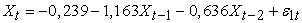(9)

And for the buying value is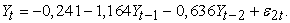(10)

Correlogram autocorrelation (ACF) and partial autocorrelation corelogram (PACF), as shown below,

• Figure 5. Residual correlogram

While the forecast value patterns are as shown below

• Figure 6. Forecast values pattern

The ARIMA model (2,2,0) for its residuals based on the results of the SPSS analysis is as follows

From the results of the analysis, it was concluded that the error model of the selling value is,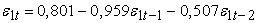(11)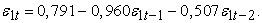(12)

### 5. Conclusion

The ARCH model for the rupiah exchange rate over US dollars is ARIMA (2,2,0), with a mathematical model for selling value isAnd for the buying value is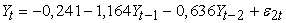This shows that there is a negative correlation between data, so the value of the rupiah exchange rate towards the US dollar is fluctuating, with a downward trend in selling and buying values.

### ReferencesThis work is licensed under a Creative Commons Attribution 4.0 International License. To view a copy of this license, visit http://creativecommons.org/licenses/by/4.0/